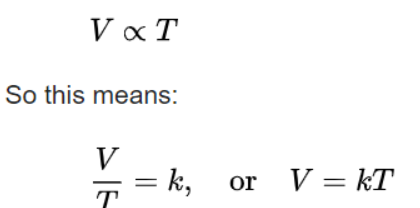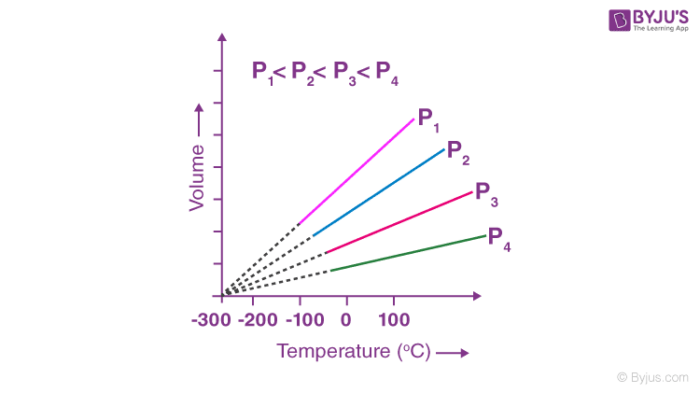# Relationship Between Temperature And Volume: Charles's Law

In 1787, the French scientist Jacques Charles discovered that the volume of a gas varies when we change its temperature, keeping the pressure constant. Later, in 1802, Joseph Gay-Lussac modified the concept given by Charles and generalized it as Charles’s law. Gases obey Charles’s law at a very high temperature and low pressure. It is a special case of the general gas law and can be derived from the kinetic theory of gases under the assumption of an ideal gas.

In simple terms, at constant pressure, the volume of a fixed mass of gas is directly proportional to temperature.Know more about Charles’s Law Formula

It can be stated as:

“The volume of a fixed mass of a gas decreases on cooling it and increases by increasing the temperature. For one degree rise in temperature, the volume of the gas increases by 1/273 of its original volume at 0˚C. Let the volume of the gas at 0˚C and t˚C be Vo and Vt respectively”.

Then,

$$\begin{array}{l}V_t = V_o + \frac{t}{273.15} V_o……….. (i)\end{array}$$
$$\begin{array}{l}V_t = V_o(1 + \frac{t}{273.15}) ……….. (ii)\end{array}$$
$$\begin{array}{l}V_t = V_o (\frac{273.15 + t}{273.15})………… (iii)\end{array}$$

We will now assign a new scale for temperature where the temperature in Celsius is given as t = T -273.15 and 0˚C can be given as To = 273.15. This new scale of temperature (T) is known as the Kelvin temperature scale or Absolute temperature scale. The degree sign is not written when a temperature is written in Kelvin scale. It is also known as the thermodynamic scale of temperature and it is commonly used for all scientific purposes. Thus, when we need to write temperature on the Kelvin scale we add 273 to the temperature in Celsius.

Let us assume T = 273.15 + t

To = 273.15

Then equation (iii) can be written as

$$\begin{array}{l}V_t = V_o(\frac{T_t}{T_o})\end{array}$$

Or,

$$\begin{array}{l}(\frac{V_t}{V_o}) = (\frac{T_t}{T_o})\end{array}$$

In general, we can write it as

$$\begin{array}{l}(\frac{V_2}{V_1}) = (\frac{T_2}{T_1})\end{array}$$

Or,

$$\begin{array}{l}(\frac{V_1}{T_1}) = (\frac{V_2}{T_2})\end{array}$$
$$\begin{array}{l} \Rightarrow \frac{V}{T} = constant = k_2\end{array}$$

Hence,

$$\begin{array}{l}V = k_{2} T\end{array}$$

The value of k2 depends on the pressure of the gas, its amount and also the unit of volume V.

Know more about Charles’s Law Calculator

Graphical representation:

At a fixed pressure, when the volume is varied, the volume-temperature relationship traces a straight line on the graph and on moving towards zero volume all lines intersect at a point on the temperature axis which is -273.15˚C. Each line in the graph of volume Vs temperature is known as isobar (Since pressure is constant). The least hypothetical temperature of -273˚C at which a gas will have zero volume is called absolute zero.In this article, we studied one of the fundamental gas laws in chemistry i.e., Charles law. To learn about other gas laws and to get deeper knowledge about the Gas Laws, download BYJU’S the learning app.’